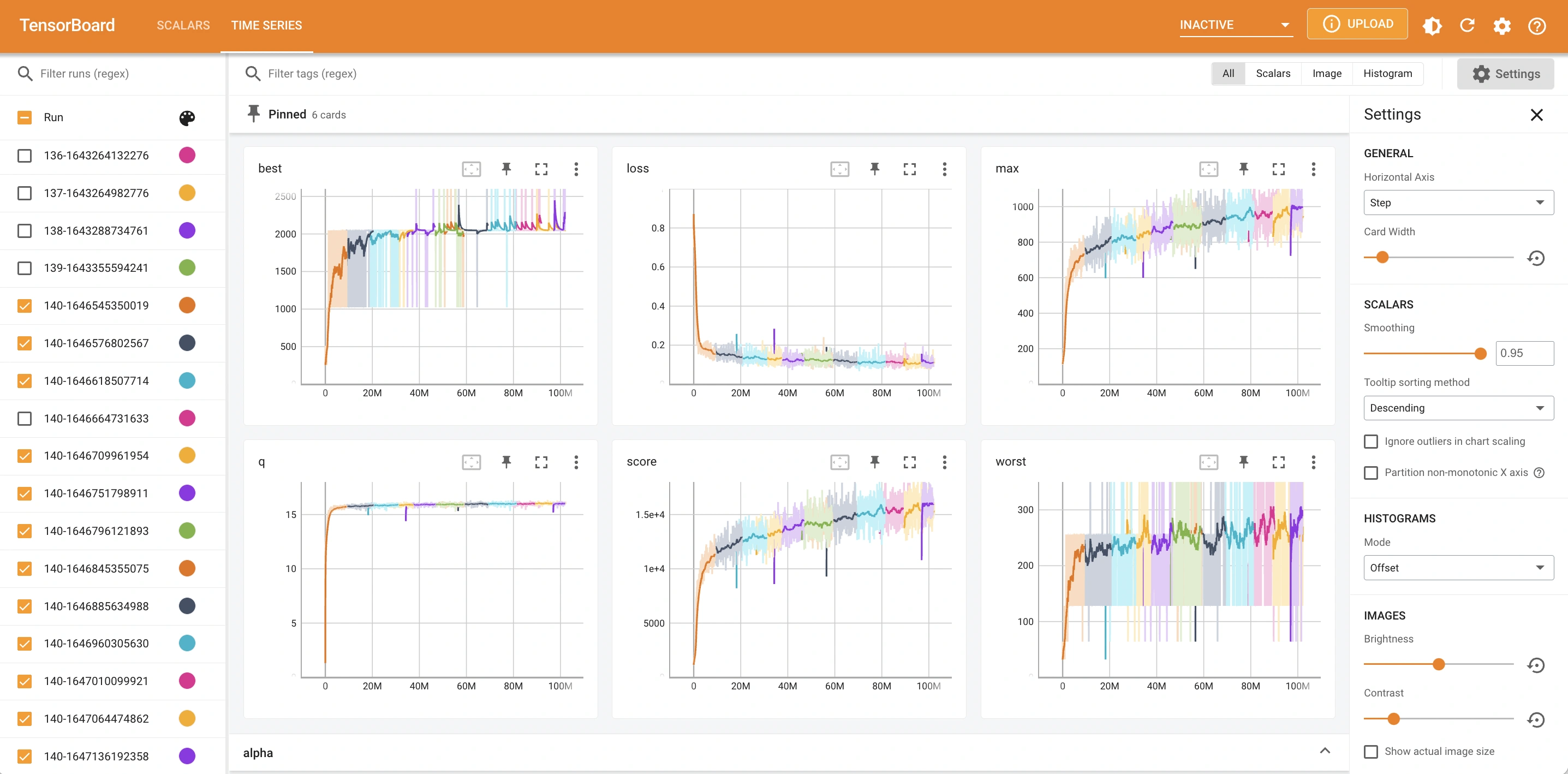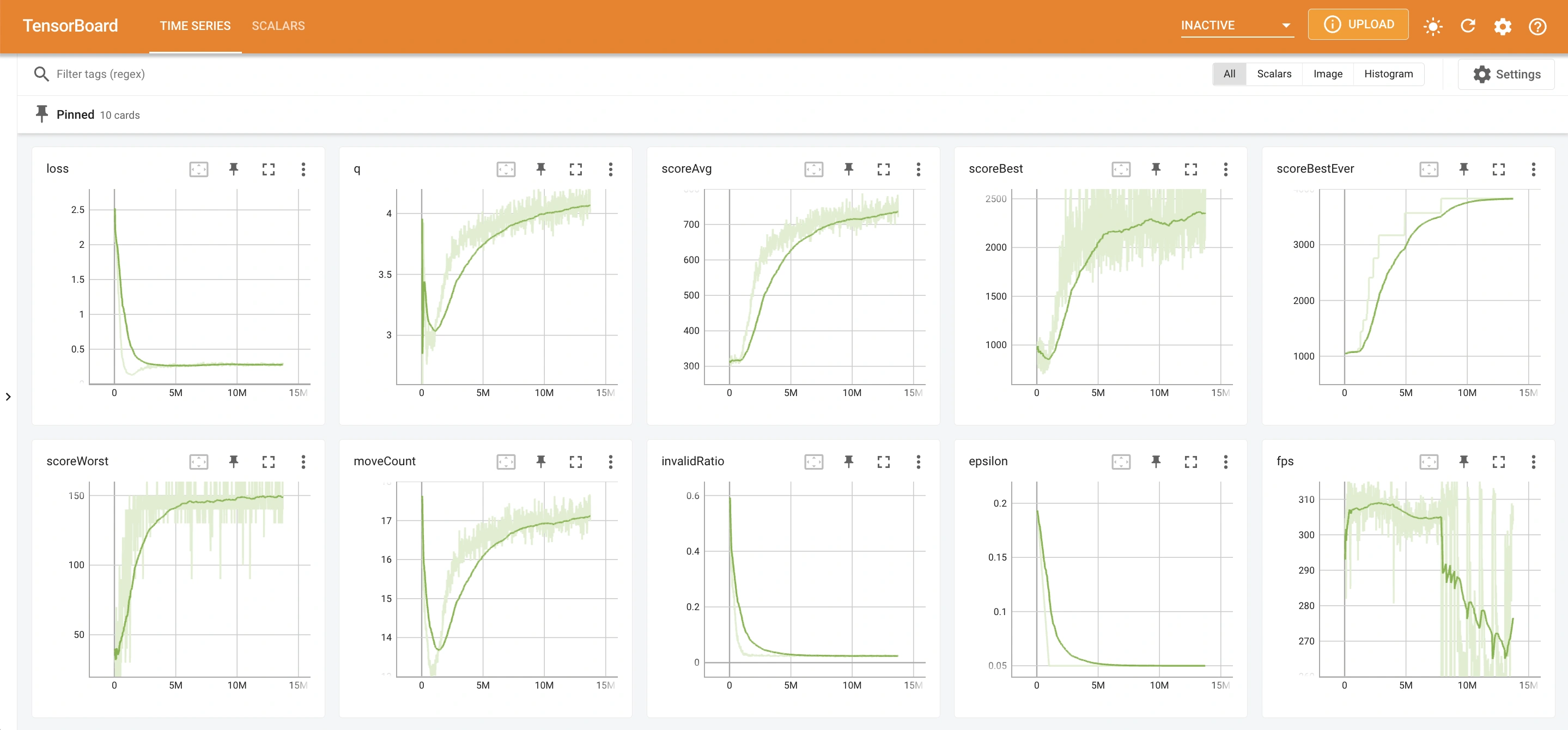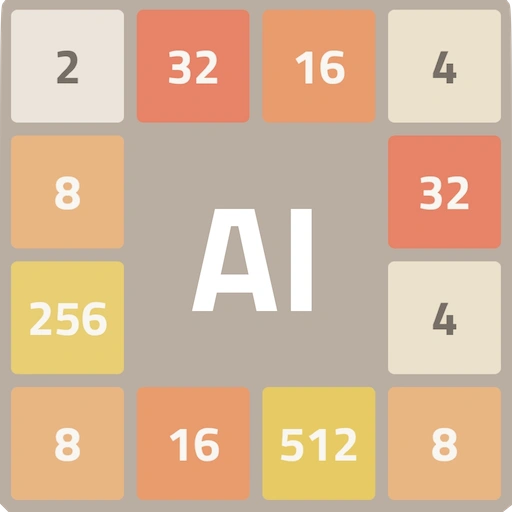Deep Q-networks (DQN) is a type of deep reinforcement learning algorithm developed by DeepMind in 2013.

DQN uses a deep convolutional neural network to approximate the Q-value of action in a given state.

### Hyperparameters

There are several hyperparameters that can be tuned to get better results with DQN.

### Performance metrics

We can use the Q value and loss as two metrics to evaluate the performance of a DQN model.

Q value

Q value measures the expected reward for performing an action in a given state.

• Increasing average Q value is a sign that model is getting better at the game (better performance).
• A good range for Q value is 5 to 20, with a small and steady increasing trend.
• It is normal for Q value to fluctuate or decrease at the start of training.

Tips for Q value

Expected Q value is affected various hyperparameters as well as the reward function.

Here are some common issues with Q values and tips on how to fix them:

1. Q value too low (<1)

• Alpha (learning rate) might be too low. Increase alpha to make the model learn faster.
• Gamma (discount factor) might be too low. Increase gamma to make the model account for more future reward.
• The model might not be learning at all. This could be due to poor design or conflicting weights in reward function.

2. Q value too high (>50)

• Gamma (discount factor) might be too high. Decrease gamma to avoid compounding future reward too much.
• Weights in reward function might be too high. Try to lower the weights for factors affecting the reward function.

3. Q value unstable and fluctuates widely

• Alpha (learning rate) might be too high. Decrease alpha to make the model learn in a more stable manner.

Loss

Loss measures the difference between the predicted and the actual result (how accurate the prediction is). It is the squared error of the target Q value and prediction Q value.

• Decreasing loss is a sign that model is becoming more accurate at predictions.
• A perfect model would have a loss value of zero, meaning it can predict state reward perfectly without any errors.
• A good range for loss value is 0 to 5, with a small and steady decreasing trend.
• It is normal for loss to increase at the start of training, before Q value stabilizes.

Tips for Loss

Here are some common issues with loss and tips on how to fix them:

1. Negative loss

• This is likely due to a bug. You can report bugs on our Discord server.

2. Loss too high (>10)

• Gamma (discount factor) might be too high. Decrease gamma to avoid compounding future reward too much.
• Weights in reward function might be too high. Try to lower the weights for factors affecting the reward function.
• The model is not learning and becoming better. This could be due to poor design or conflicting weights in reward function.

3. Loss unstable and fluctuates widely

• Alpha (learning rate) might be too high. Decrease alpha to make the model learn in a more stable manner.

### Examples

DQN can be trained to play many single-player games, for example Tetris, Snake, 2048.

This is a screenshot of tfboard for training DQN to play AI Simulator: 2048 over 100M frames:Observations on key metrics:

• The Q value is stable around 15 to 16 and increasing steadily.
• The loss value is stable at around 0.1 to 0.2 and decreasing steadily.

This is a screenshot of tfboard for training DQN to play AI Simulator: Robot over 13M frames:Observations on key metrics:

• The Q value is stable around 4 and increasing steadily.
• The loss value is stable at around 0.3 and decreasing steadily.

DQN paper

### Interactive demos

Download AI Simulator games to try using DQN algorithm on the various games:# AI Simulator: 2048

Play with numbers
Train machine learning models
See the stats grow# AI Simulator: Robot

Navigate maze with AI